# Inverse function

The function that associates with each of the elements of the set of values of a given function the set of all elements from the domain of definition of the given function that are mapped onto it, that is, its complete inverse image. If the given function is denoted by, then the inverse function is denoted by. Thus, ifandis the range of,, then for any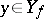one has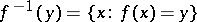.
If for allthe complete inverse image ofconsists of precisely one element, that is, if the mapping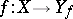is a bijection, then the inverse function is single-valued, otherwise it is many-valued.
If the setsandare subsets of the real line (or, more generally, of some ordered sets) then strict monotonicity ofis a necessary and sufficient condition for the existence of an inverse function that is also strictly monotone.
A number of properties of the inverse function can be determined from the corresponding properties of. For example, ifis strictly monotone and continuous on some interval of the real line, then its inverse is also monotone and continuous on the corresponding interval. If a one-to-one mapping of a compactum onto a Hausdorff topological space is continuous, then the inverse mapping is also continuous. That is, the original mapping is a homeomorphism onto its image. When the mappingis a one-to-one bounded linear operator mapping a Banach spaceonto a Banach space, then the inverse operatoris also linear and bounded.
Letbe a continuous mapping of the closure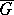of a bounded domain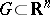,, with a sufficiently smooth boundary in, letbe differentiable inand map the boundary ofonto the boundary ofand suppose that the set of zeros of the Jacobian ofis an isolated set; then ifis one-to-one on the boundary of, it is one-to-one on. For the existence of a local inverse mapping in a neighbourhood of a given point it is sufficient that the Jacobian of the mapping does not vanish in some neighbourhood of this point. If,, is a differentiable mapping with non-zero Jacobian at all points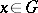, then for any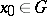there exists a neighbourhood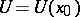such that the restriction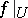oftois a one-to-one mapping ofonto some neighbourhoodof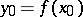, and the inverse mapping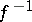is also differentiable (on). This theorem can be generalized to the infinite-dimensional case: Letandbe complete normed spaces, letbe an open set and letbe a continuously-differentiable mapping. If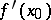is an invertible element in the space of bounded linear operators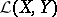(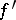is the Fréchet derivative),, then there exists neighbourhoodsand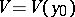ofandinandrespectively, such that the mapping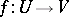and its inverse mapping are continuously-differentiable homeomorphisms.